Skip to content →

# Tag: symmetry

We are after the geometric trinity corresponding to the trinity of exceptional Galois groupsThe surfaces on the right have the corresponding group on the left as their group of automorphisms. But, there is a lot more group-theoretic info hidden in the geometry. Before we sketch the $L_2(11)$ case, let us recall the simpler situation of $L_2(7)$.

There are some excellent web-page on the Klein quartic and it would be too hard to try to improve on them, so we refer to John Baez’ page and Greg Egan’s page for more details.

The Klein quartic is the degree 4 projective plane curve defined by the equation $x^3y+y^3z+z^3x=0$. It can be tiled with a set of 24 regular heptagons, or alternatively with a set of 56 equilateral triangles and these two tilings are dual to each other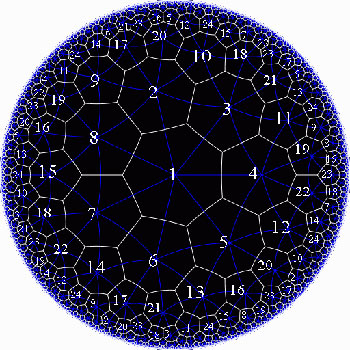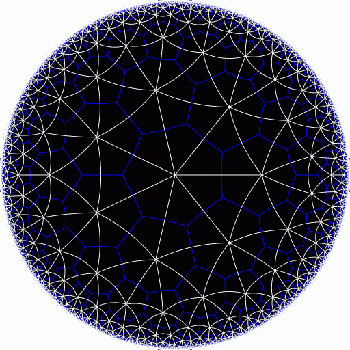In the triangular tiling, there are 56 triangles, 84 edges and 24 vertices. The 56 triangles come in 7 bunches of 8 each and we give the 7 bunches of triangles each a different color as in the pictures below made by Greg Egan. Observe that in the hyperbolic tiling all triangles look alike, but in the picture on the left most of them get warped as we try to embed the quartic in 3-space (which is impossible to do properly). The non-warped triangles (the red ones) come into pairs, the top and bottom triangles of a triangular prism, one prism at each of the four ‘vertices’ of a tetrahedron.

The automorphism group $L_2(7)$ acts on these triangles as $S_4$ acts on the triangles in a truncated cube.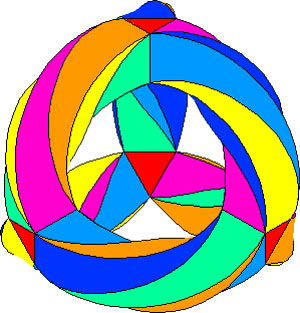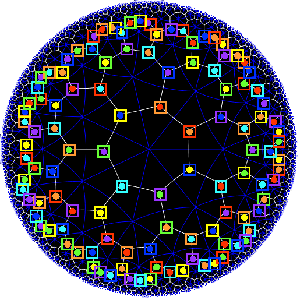The buckyball construction from a conjugacy class of order 11 elements from $L_2(11)$ recalled last time, has an analogon $L_2(7)$, leading to the truncated cube.

In $L_2(7)$ there are two conjugacy classes of subgroups isomorphic to $S_4$ (the rotation-symmetry group of the cube) as well as two conjugacy classes of order 7 elements, each consisting of precisely 24 elements, say C and D. The normalizer subgroup of C has order 21, so there is a cyclic group of order 3 acting non-trivially on the conjugacy class C with 8 orbits consisting of three elements each. These are the eight triangles of the truncated cube identified above as the red triangles.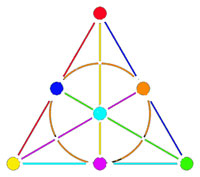Shifting perspective, we can repeat this for each of the seven different colors. That is, we have seven truncated cubes in the Klein quartic. On each of them a copy of $S_4$ acts and these subgroups form one of the two conjugacy classes of $S_4$ in the group $L_2(7)$. The colors of the triangles of these seven truncated cubes are indicated by bullets in the picture above on the right. The other conjugacy class of $S_4$’s act on ‘truncated anti-cubes’ which also come in seven bunches of which the color is indicated by a square in that picture.

If you spend enough time on it you will see that each (truncated) cube is completely disjoint from precisely 3 (truncated) anti-cubes. This reminds us of the Fano-plane (picture on the left) : it has 7 points (our seven truncated cubes), 7 lines (the truncated anti-cubes) and the incidence relation of points and lines corresponds to the disjointness of (truncated) cubes and anti-cubes! This is the geometric interpretation of the group-theoretic realization that $L_2(7) \simeq PGL_3(\mathbb{F}_2)$ is the isomorphism group of the projective plane over the finite field $\mathbb{F}_2$ on two elements, that is, the Fano plane. The colors of the picture on the left indicate the colors of cubes (points) and anti-cubes (lines) consistent with Egan’s picture above.

Further, the 24 vertices correspond to the 24 cusps of the modular group $\Gamma(7)$. Recall that a modular interpretation of the Klein quartic is as $\mathbb{H}/\Gamma(7)$ where $\mathbb{H}$ is the upper half-plane on which the modular group $\Gamma = PSL_2(\mathbb{Z})$ acts via Moebius transformations, that is, to a 2×2 matrix corresponds the transformation

$$\begin{bmatrix} a & b \\ c & d \end{bmatrix}$$ <----> $z \mapsto \frac{az+b}{cz+d}$

Okay, now let’s briefly sketch the exciting results found by Pablo Martin and David Singerman in the paper From biplanes to the Klein quartic and the buckyball, extending the above to the group $L_2(11)$.

There is one important modification to be made. Recall that the Cayley-graph to get the truncated cube comes from taking as generators of the group $S_4$ the set ${ (3,4),(1,2,3) }$, that is, an order two and an order three element, defining an epimorphism from the modular group $\Gamma= C_2 \ast C_3 \rightarrow S_4$.

We have also seen that in order to get the buckyball as a Cayley-graph for $A_5$ we need to take the generating set ${ (2,3)(4,5),(1,2,3,4,5) }$, so a degree two and a degree five element.

Hence, if we want to have a corresponding Riemann surface we’d better not start from the action of the modular group on the upper half-plane, but rather the action via Moebius transformations of the
Hecke group

$H^5 \simeq C_2 \ast C_5 = \langle z \mapsto -\frac{1}{z}, z \mapsto z+ \phi \rangle$

where $\phi = \frac{1 + \sqrt{5}}{2}$ is the golden ratio.

But then, there is an epimorphism $H^5 \rightarrow L_2(11)$ (as this group is generated by one element of degree 2 and one of degree 5) and let $\Lambda$ denote its kernel. Observe that $\Lambda$ is the analogon of the modular subgroup $\Gamma(7)$ used above to define the Klein quartic.

Hence, Martin and Singerman define the buckyball curve as the modular quotient $X=\mathbb{H}/\Lambda$ which is a Riemann surface of genus 70.

The terminlogy is motivated by the fact that, precisely as we got 7 truncated cubes in the Klein quartic, we now get 11 truncated icosahedra (that is, buckyballs) in $X$. The 11 coming, analogous to the Klein case, from thefact that there are precisely two conjugacy classes of subgroups of $L_2(11)$ isomorphic to $A_5$, each class containing precisely eleven elements!
The 60 vertices of the buckyball again correspond to the fact that there are 60 cusps in this case.

So, what is the analogon of the Fano plane in this case? Well, observe that the Fano-plane is a biplane of order two. That is, if we take as ‘points’ the points of the Fano plane and as ‘lines’ the complements of lines in the Fano plane then this defines a biplane structure. This means that any two distinct ‘points’ are contained in two distinct ‘lines’ and that two distinct ‘lines’ intersect in two distinct ‘points’. A biplane is said to be of order k is each ‘line’ consist of k-2 ‘points’. As the complement of a line in the Fano plane consists of 4 points, the Fano plane is therefore a biplane of order 2. The intersection pattern of cubes and anti-cubes in the Klein quartic is this biplane structure on the Fano plane.

In a similar way, Martin and Singerman show that the two conjugacy classes of subgroups isomorphic to $A_5$ in $L_2(11)$, each containing exactly 11 elements, correspond to 11 embedded buckyballs (and 11 anti-buckyballs) in the buckyball-curve $X$ and that the intersection relations among them describe the combinatorial structure of a biplane of order three if we view the 11 buckys as ‘points’ and the anti-buckys as ‘lines’.

That is, the buckyball curve is a perfect geometric counterpart of the Klein quartic for the two trinitiesAt the Arcadian Functor, Kea also has a post on this in which she conjectures that the Kac-Moody algebra of E11 may be related to the buckyball curve.

References :

David Singerman, “Klein’s Riemann surface of genus 3 and regular embeddings of finite projective planes” Bull. London Math. Soc. 18 (1986) 364-370.

Pablo Martin and David Singerman, “From biplanes to the Klein quartic and the Buckyball” (note that this is a preliminary version, please contact David Singerman for the latest version).

The buckyball is without doubt the hottest mahematical object at the moment (at least in Europe). Recall that the buckyball (middle) is a mixed form of two Platonic solids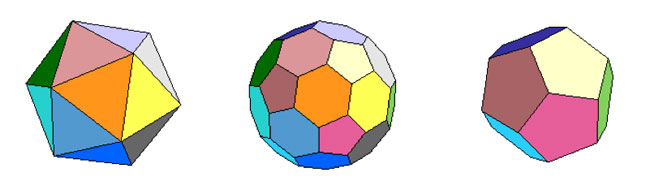the Icosahedron on the left and the Dodecahedron on the right.

For those of you who don’t know anything about football, it is that other ball-game, best described via a quote from the English player Gary Lineker

“Football is a game for 22 people that run around, play the ball, and one referee who makes a slew of mistakes, and in the end Germany always wins.”

We still have a few days left hoping for a better ending… Let’s do some bucky-maths : what is the rotation symmetry group of the buckyball?

For starters, dodeca- and icosahedron are dual solids, meaning that if you take the center of every face of a dodecahedron and connect these points by edges when the corresponding faces share an edge, you’ll end up with the icosahedron (and conversely). Therefore, both solids (as well as their mixture, the buckyball) will have the same group of rotational symmetries. Can we at least determine the number of these symmetries?

Take the dodecahedron and fix a face. It is easy to find a rotation taking this face to anyone of its five adjacent faces. In group-slang : the rotation automorphism group acts transitively on the 12 faces of the dodecohedron. Now, how many of them fix a given face? These can only be rotations with axis through the center of the face and there are exactly 5 of them preserving the pentagonal face. So, in all we have $12 \times 5 = 60$ rotations preserving any of the three solids above. By composing two of its elements, we get another rotational symmetry, so they form a group and we would like to determine what that group is.

There is one group that springs to mind $A_5$, the subgroup of all even permutations on 5 elements. In general, the alternating group has half as many elements as the full permutation group $S_n$, that is $\frac{1}{2} n!$ (for multiplying with the involution (1,2) gives a bijection between even and odd permutations). So, for $A_5$ we get 60 elements and we can list them :

• the trivial permutation$~()$, being the identity.
• permutations of order two with cycle-decompostion $~(i_1,i_2)(i_3,i_4)$, and there are exactly 15 of them around when all numbers are between 1 and 5.
• permutations of order three with cycle-form $~(i_1,i_2,i_3)$ of which there are exactly 20.
• permutations of order 5 which have to form one full cycle $~(i_1,i_2,i_3,i_4,i_5)$. There are 24 of those.

Can we at least view these sets of elements as rotations of the buckyball? Well, a dodecahedron has 12 pentagobal faces. So there are 4 nontrivial rotations of order 5 for every 2 opposite faces and hence the dodecaheder (and therefore also the buckyball) has indeed 6×4=24 order 5 rotational symmetries.

The icosahedron has twenty triangles as faces, so any of the 10 pairs of opposite faces is responsible for two non-trivial rotations of order three, giving us 10×2=20 order 3 rotational symmetries of the buckyball.

The order two elements are slightly harder to see. The icosahedron has 30 edges and there is a plane going through each of the 15 pairs of opposite edges splitting the icosahedron in two. Hence rotating to interchange these two edges gives one rotational symmetry of order 2 for each of the 15 pairs.

And as 24+20+15+1(identity) = 60 we have found all the rotational symmetries and we see that they pair up nicely with the elements of $A_5$. But do they form isomorphic groups? In other words, can the buckyball see the 5 in the group $A_5$.

In a previous post I’ve shown that one way to see this 5 is as the number of inscribed cubes in the dodecahedron. But, there is another way to see the five based on the order 2 elements described before.

If you look at pairs of opposite edges of the icosahedron you will find that they really come in triples such that the planes determined by each pair are mutually orthogonal (it is best to feel this on ac actual icosahedron). Hence there are 15/3 = 5 such triples of mutually orthogonal symmetry planes of the icosahedron and of course any rotation permutes these triples. It takes a bit of more work to really check that this action is indeed the natural permutation action of $A_5$ on 5 elements.

Having convinced ourselves that the group of rotations of the buckyball is indeed the alternating group $A_5$, we can reverse the problem : can the alternating group $A_5$ see the buckyball???

Well, for starters, it can ‘see’ the icosahedron in a truly amazing way. Look at the conjugacy classes of $A_5$. We all know that in the full symmetric group $S_n$ elements belong to the same conjugacy class if and only if they have the same cycle decomposition and this is proved using the fact that the conjugation f a cycle $~(i_1,i_2,\ldots,i_k)$ under a permutation $\sigma \in S_n$ is equal to the cycle $~(\sigma(i_1),\sigma(i_2),\ldots,\sigma(i_k))$ (and this gives us also the candidate needed to conjugate two partitions into each other).

Using this trick it is easy to see that all the 15 order 2 elements of $A_5$ form one conjugacy class, as do the 20 order 3 elements. However, the 24 order 5 elements split up in two conjugacy classes of 12 elements as the permutation needed to conjugate $~(1,2,3,4,5)$ to $~(1,2,3,5,4)$ is $~(4,5)$ but this is not an element of $A_5$.

Okay, now take one of these two conjugacy classes of order 5 elements, say that of $~(1,2,3,4,5)$. It consists of 12 elements, 12 being also the number of vertices of the icosahedron. So, is there a way to identify the elements in the conjugacy class to the vertices in such a way that we can describe the edges also in terms of group-computations in $A_5$?

Surprisingly, this is indeed the case as is demonstrated in a marvelous paper by Kostant “The graph of the truncated icosahedron and the last letter of Galois”.

Two elements $a,b$ in the conjugacy class C share an edge if and only if their product $a.b \in A_5$ still belongs to the conjugacy class C!

So, for example $~(1,2,3,4,5).(2,1,4,3,5) = (2,5,4)$ so there is no edge between these elements, but on the other hand $~(1,2,3,4,5).(5,3,4,1,2)=(1,5,2,4,3)$ so there is an edge between these! It is no coincidence that $~(5,3,4,1,2)=(2,1,4,3,5)^{-1}$ as inverse elements correspond in the bijection to opposite vertices and for any pair of non-opposite vertices of an icosahedron it is true that either they are neighbors or any one of them is the neighbor of the opposite vertex of the other element.

If we take $u=(1,2,3,4,5)$ and $v=(5,3,4,1,2)$ (or any two elements of the conjugacy class such that u.v is again in the conjugacy class), then one can describe all the vertices of the icosahedron group-theoretically as follows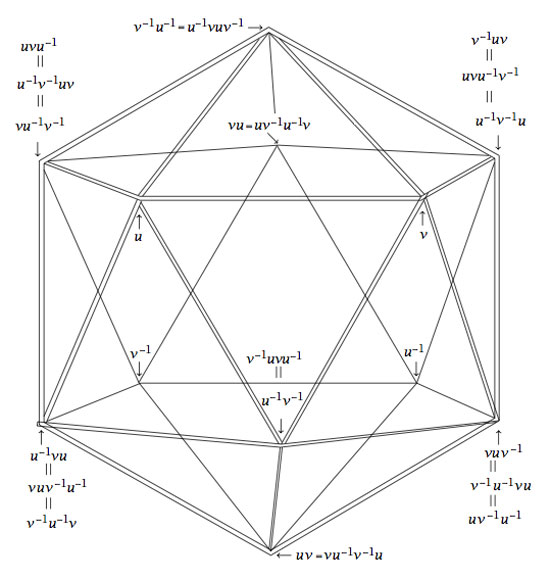Isn’t that nice? Well yes, you may say, but that is just the icosahedron. Can the group $A_5$ also see the buckyball?

Well, let’s try a similar strategy : the buckyball has 60 vertices, exactly as many as there are elements in the group $A_5$. Is there a way to connect certain elements in a group according to fixed rules? Yes, there is such a way and it is called the Cayley Graph of a group. It goes like this : take a set of generators ${ g_1,\ldots,g_k }$ of a group G, then connect two group element $a,b \in G$ with an edge if and only if $a = g_i.b$ or $b = g_i.a$ for some of the generators.

Back to the alternating group $A_5$. There are several sets of generators, one of them being the elements ${ (1,2,3,4,5),(2,3)(4,5) }$. In the paper mentioned before, Kostant gives an impressive group-theoretic proof of the fact that the Cayley-graph of $A_5$ with respect to these two generators is indeed the buckyball!

Let us allow to be lazy for once and let SAGE do the hard work for us, and let us just watch the outcome. Here’s how that’s done

A=PermutationGroup([‘(1,2,3,4,5)’,'(2,3)(4,5)’])
B=A.cayley_graph()
B.show3d()

The outcone is a nice 3-dimensional picture of the buckyball. Below you can see a still, and, if you click on it you will get a 3-dimensional model of it (first click the ‘here’ link in the new window and then you’d better control-click and set the zoom to 200% before you rotate it)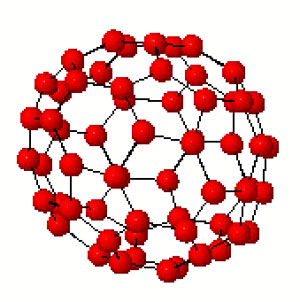Hence, viewing this Cayley graph from different points we have convinced ourselves that it is indeed the buckyball. In fact, most (truncated) Platonic solids appear as Cayley graphs of groups with respect to specific sets of generators. For later use here is a (partial) survey (taken from Jaap’s puzzle page)Tetrahedron : $C_2 \times C_2,[(12)(34),(13)(24),(14)(23)]$
Cube : $D_4,[(1234),(13)]$
Octahedron : $S_3,[(123),(12),(23)]$
Dodecahedron : IMPOSSIBLE
Icosahedron : $A_4,[(123),(234),(13)(24)]$Truncated tetrahedron : $A_4,[(123),(12)(34)]$
Cuboctahedron : $A_4,[(123),(234)]$
Truncated cube : $S_4,[(123),(34)]$
Truncated octahedron : $S_4,[(1234),(12)]$
Rhombicubotahedron : $S_4,[(1234),(123)]$
Rhombitruncated cuboctahedron : IMPOSSIBLE
Snub cuboctahedron : $S_4,[(1234),(123),(34)]$Icosidodecahedron : IMPOSSIBLE
Truncated dodecahedron : $A_5,[(124),(23)(45)]$
Truncated icosahedron : $A_5,[(12345),(23)(45)]$
Rhombicosidodecahedron : $A_5,[(12345),(124)]$
Rhombitruncated icosidodecahedron : IMPOSSIBLE
Snub Icosidodecahedron : $A_5,[(12345),(124),(23)(45)]$

Again, all these statements can be easily verified using SAGE via the method described before. Next time we will go further into the Kostant’s group-theoretic proof that the buckyball is the Cayley graph of $A_5$ with respect to (2,5)-generators as this calculation will be crucial in the description of the buckyball curve, the genus 70 Riemann surface discovered by David Singerman and
Pablo Martin which completes the trinity corresponding to the Galois trinityArnold has written a follow-up to the paper mentioned last time called “Polymathematics : is mathematics a single science or a set of arts?” (or here for a (huge) PDF-conversion).

On page 8 of that paper is a nice summary of his 25 trinities :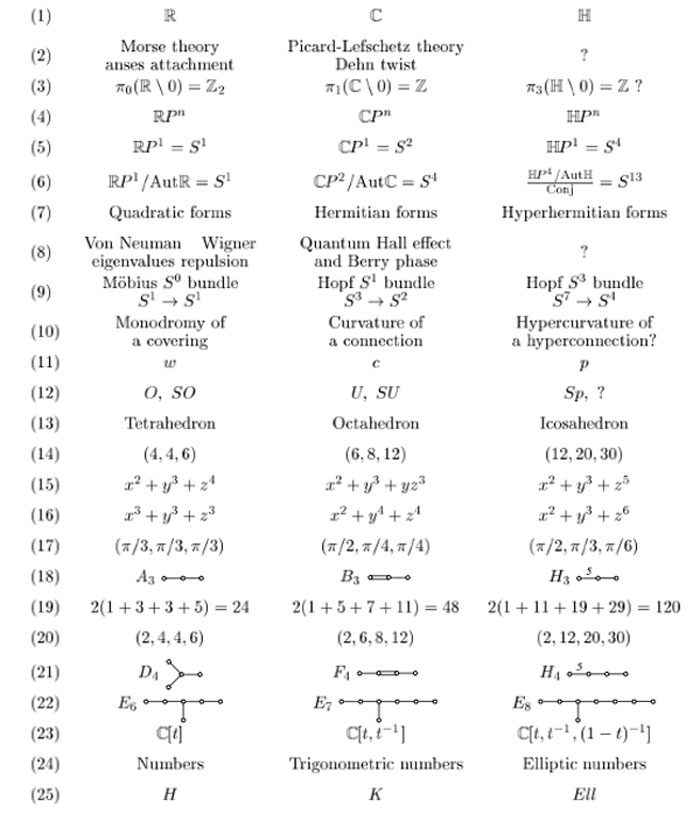I learned of this newer paper from a comment by Frederic Chapoton who maintains a nice webpage dedicated to trinities.

In his list there is one trinity on sporadic groups :where $F_{24}$ is the Fischer simple group of order $2^{21}.3^{16}.5^2.7^3.11.13.17.23.29 = 1255205709190661721292800$, which is the third largest sporadic group (the two larger ones being the Baby Monster and the Monster itself).

I don’t know what the rationale is behind this trinity. But I’d like to recall the (Baby)Monster history as a warning against the trinity-reflex. Sometimes, there is just no way to extend a would be trinity.

The story comes from Mark Ronan’s book Symmetry and the Monster on page 178.

Let’s remind ourselves how we got here. A few years earlier, Fischer has created his ‘transposition’ groups Fi22, Fi23, and Fi24. He had called them M(22), M(23), and M(24), because they were related to Mathieu’s groups M22,M23, and M24, and since he used Fi22 to create his new group of mirror symmetries, he tentatively called it $M^{22}$.
It seemed to appear as a cross-section in something even bigger, and as this larger group was clearly associated with Fi24, he labeled it $M^{24}$. Was there something in between that could be called $M^{23}$?
Fischer visited Cambridge to talk on his new work, and Conway named these three potential groups the Baby Monster, the Middle Monster, and the Super Monster. When it became clear that the Middle Monster didn’t exist, Conway settled on the names Baby Monster and Monster, and this became the standard terminology.

Marcus du Sautoy’s account in Finding Moonshine is slightly different. He tells on page 322 that the Super Monster didn’t exist. Anyone knowing the factual story?

Some mathematical trickery later revealed that the Super Monster was going to be impossible to build: there were certain features that contradicted each other. It was just a mirage, which vanished under closer scrutiny. But the other two were still looking robust. The Middle Monster was rechristened simply the Monster.

And, the inclusion diagram of the sporadic simples tells yet another story.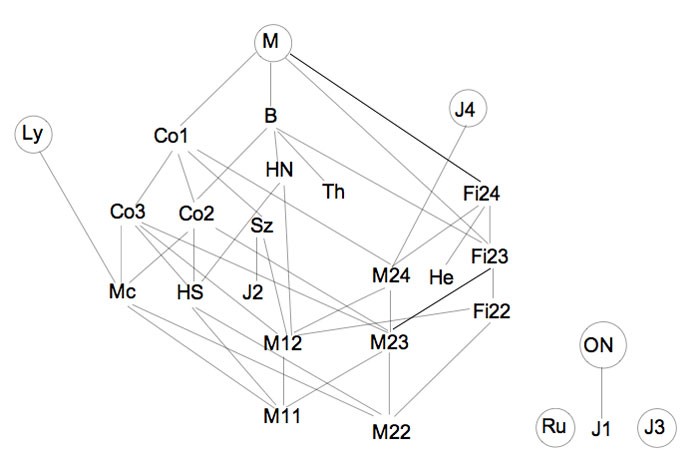Anyhow, this inclusion diagram is helpful in seeing the three generations of the Happy Family (as well as the Pariahs) of the sporadic groups, terminology invented by Robert Griess in his 100+p Inventiones paper on the construction of the Monster (which he liked to call, for obvious reasons, the Friendly Giant denoted by FG).
The happy family appears in Table 1.1. of the introduction.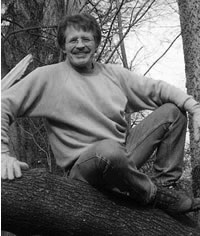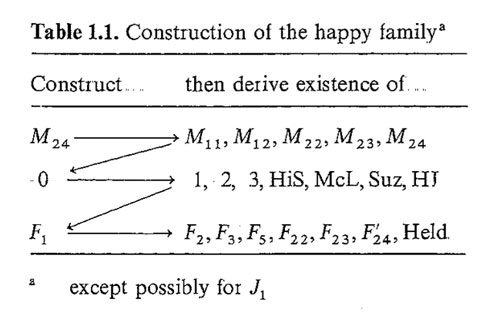It was this picture that made me propose the trinity on the left below in the previous post. I now like to add another trinity on the right, and, the connection between the two is clear.Here $Golay$ denotes the extended binary Golay code of which the Mathieu group $M_{24}$ is the automorphism group. $Leech$ is of course the 24-dimensional Leech lattice of which the automorphism group is a double cover of the Conway group $Co_1$. $Griess$ is the Griess algebra which is a nonassociative 196884-dimensional algebra of which the automorphism group is the Monster.

I am aware of a construction of the Leech lattice involving the quaternions (the icosian construction of chapter 8, section 2.2 of SPLAG). Does anyone know of a construction of the Griess algebra involving octonions???Referring to the triple of exceptional Galois groups $L_2(5),L_2(7),L_2(11)$ and its connection to the Platonic solids I wrote : “It sure seems that surprises often come in triples…”. Briefly I considered replacing triples by trinities, but then, I didnt want to sound too mystic…

David Corfield of the n-category cafe and a dialogue on infinity (and perhaps other blogs I’m unaware of) pointed me to the paper Symplectization, complexification and mathematical trinities by Vladimir I. Arnold. (Update : here is a PDF-conversion of the paper)

The paper is a write-up of the second in a series of three lectures Arnold gave in june 1997 at the meeting in the Fields Institute dedicated to his 60th birthday. The goal of that lecture was to explain some mathematical dreams he had.

The next dream I want to present is an even more fantastic set of theorems and conjectures. Here I also have no theory and actually the ideas form a kind of religion rather than mathematics.
The key observation is that in mathematics one encounters many trinities. I shall present a list of examples. The main dream (or conjecture) is that all these trinities are united by some rectangular “commutative diagrams”.
I mean the existence of some “functorial” constructions connecting different trinities. The knowledge of the existence of these diagrams provides some new conjectures which might turn to be true theorems.

Follows a list of 12 trinities, many taken from Arnold’s field of expertise being differential geometry. I’ll restrict to the more algebraically inclined ones.

1 : “The first trinity everyone knows is”where $\mathbb{H}$ are the Hamiltonian quaternions. The trinity on the left may be natural to differential geometers who see real and complex and hyper-Kaehler manifolds as distinct but related beasts, but I’m willing to bet that most algebraists would settle for the trinity on the right where $\mathbb{O}$ are the octonions.

2 : The next trinity is that of the exceptional Lie algebras E6, E7 and E8.with corresponding Dynkin-Coxeter diagramsArnold has this to say about the apparent ubiquity of Dynkin diagrams in mathematics.

Manin told me once that the reason why we always encounter this list in many different mathematical classifications is its presence in the hardware of our brain (which is thus unable to discover a more complicated scheme).
I still hope there exists a better reason that once should be discovered.

Amen to that. I’m quite hopeful human evolution will overcome the limitations of Manin’s brain…

3 : Next comes the Platonic trinity of the tetrahedron, cube and dodecahedronClearly one can argue against this trinity as follows : a tetrahedron is a bunch of triangles such that there are exactly 3 of them meeting in each vertex, a cube is a bunch of squares, again 3 meeting in every vertex, a dodecahedron is a bunch of pentagons 3 meeting in every vertex… and we can continue the pattern. What should be a bunch a hexagons such that in each vertex exactly 3 of them meet? Well, only one possibility : it must be the hexagonal tiling (on the left below). And in normal Euclidian space we cannot have a bunch of septagons such that three of them meet in every vertex, but in hyperbolic geometry this is still possible and leads to the Klein quartic (on the right). Check out this wonderful post by John Baez for more on this.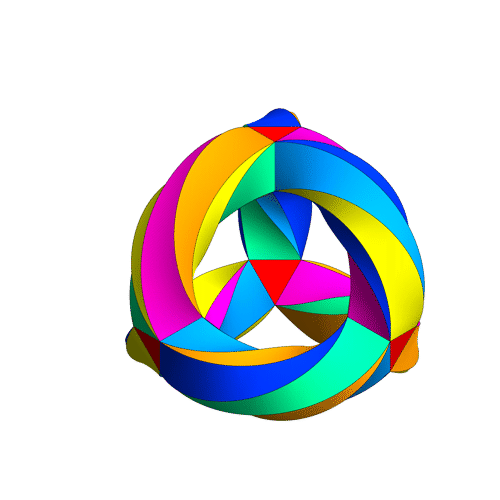4 : The trinity of the rotation symmetry groups of the three Platonicswhere $A_n$ is the alternating group on n letters and $S_n$ is the symmetric group.

Clearly, any rotation of a Platonic solid takes vertices to vertices, edges to edges and faces to faces. For the tetrahedron we can easily see the 4 of the group $A_4$, say the 4 vertices. But what is the 4 of $S_4$ in the case of a cube? Well, a cube has 4 body-diagonals and they are permuted under the rotational symmetries. The most difficult case is to see the $5$ of $A_5$ in the dodecahedron. Well, here’s the solution to this riddle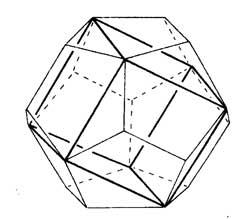there are exactly 5 inscribed cubes in a dodecahedron and they are permuted by the rotations in the same way as $A_5$.

7 : The seventh trinity involves complex polynomials in one variablethe Laurant polynomials and the modular polynomials (that is, rational functions with three poles at 0,1 and $\infty$.

8 : The eight one is another beautyHere ‘numbers’ are the ordinary complex numbers $\mathbb{C}$, the ‘trigonometric numbers’ are the quantum version of those (aka q-numbers) which is a one-parameter deformation and finally, the ‘elliptic numbers’ are a two-dimensional deformation. If you ever encountered a Sklyanin algebra this will sound familiar.

This trinity is based on a paper of Turaev and Frenkel and I must come back to it some time…

The paper has some other nice trinities (such as those among Whitney, Chern and Pontryagin classes) but as I cannot add anything sensible to it, let us include a few more algebraic trinities. The first one attributed by Arnold to John McKay

13 : A trinity parallel to the exceptional Lie algebra one isbetween the 27 straight lines on a cubic surface, the 28 bitangents on a quartic plane curve and the 120 tritangent planes of a canonic sextic curve of genus 4.

14 : The exceptional Galois groupsexplained last time.

15 : The associated curves with these groups as symmetry groups (as in the previous post)where the ? refers to the mysterious genus 70 curve. I’ll check with one of the authors whether there is still an embargo on the content of this paper and if not come back to it in full detail.

16 : The three generations of sporadic groupsDo you have other trinities you’d like to worship?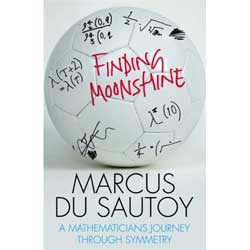On friday, I did spot in my regular Antwerp-bookshop Finding Moonshine by Marcus du Sautoy and must have uttered a tiny curse because, at once, everyone near me was staring at me…

To make matters worse, I took the book from the shelf, quickly glanced through it and began shaking my head more and more, the more I convinced myself that it was a mere resampling of Symmetry and the Monster, The equation that couldn’t be solved, From Error-Correcting Codes through Sphere Packings to Simple Groups and the diary-columns du Sautoy wrote for a couple of UK-newspapers about his ‘life-as-a-mathematician’…

Still, I took the book home, made a pot of coffee and started reading the first chapter. And, sure enough, soon I had to read phrases like “The first team consisted of a ramshackle collection of mathematical mavericks. One of the most colourful was John Horton Conway, currently professor at the University of Princeton. His mathematical and personal charisma have given him almost cult status…” and “Conway, the Long John Silver of mathematics, decided that an account should be published of the lands that they had discovered on their voyage…” and so on, and so on, and so on.

The main problem I have with du Sautoy’s books is that their main topic is NOT mathematics, but rather the lives of mathematicians (colourlful described with childlike devotion) and the prestige of mathematical institutes (giving the impression that it is impossible to do mathematics of quality if one isn’t living in Princeton, Paris, Cambridge, Bonn or … Oxford). Less than a month ago, I reread his ‘Music of the Primes’ so all these phrases were still fresh in my memory, only on that occasion Alain Connes is playing Conway’s present role…

I was about to throw the book away, but first I wanted to read what other people thought about it. So, I found Timothy Gowers’ review, dated febraury 21st, in the Times Higher Education. The first paragraph below hints politely at the problems I had with Music of the Primes, but then, his conclusion was a surprise

The attitude of many professional mathematicians to the earlier book was ambivalent. Although they were pleased that du Sautoy was promoting mathematics, they were not always convinced by the way that he did it.

I myself expected to have a similar attitude to Finding Moonshine, but du Sautoy surprised me: he has pulled off that rare feat of writing in a way that can entertain and inform two different audiences – expert and non-expert – at the same time.

Okay, so maybe I should give ‘Finding Moonshine’ a further chance. After all, it is week-end and, I have nothing else to do than attending two family-parties… so I read the entire book in a couple of hours (not that difficult to do if you skip all paragraphs that have the look and feel of being copied from the books mentioned above) and, I admit, towards the end I mellowed a bit. Reading his diary notes I even felt empathy at times (if this is possible as du Sautoy makes a point of telling the world that most of us mathematicians are Aspergers). One example :

One of my graduate students has just left my office. He’s done some great work over the past three years and is starting to write up his doctorate, but he’s just confessed that he’s not sure that he wants to be a mathematician. I’m feeling quite sobered by this news. My graduate students are like my children. They are the future of the subject. Who’s going to read all the details of my papers if not my mathematical offspring? The subject feels so tribal that anyone who says they want out is almost a threat to everything the tribe stands for.
Anton has been working on a project very close to my current problem. There’s no denying that one can feel quite disillusioned by not finding a way into a problem. Last year one of my post-docs left for the City after attempting to scale this mountain with me. I’d already rescued him from being dragged off to the City once before. But after battling with our problem and seeing it become more and more complex, he felt that he wasn’t really cut out for it.

What is unsettling for me is that they both questioned the importance of what we are doing. They’ve asked that ‘What’s it all for?’ question, and think they’ve seen the Emperor without any clothes.

Anton has questioned whether the problems we are working on are really important. I’ve explained why I think these are fundamental questions about basic objects in nature, but I can see that he isn’t convinced. I feel I am having to defend my whole existence. I’ve arranged for him to join me at a conference in Israel later this month, and I hope that seeing the rest of the tribe enthused and excited about these problems will re-inspire him. It will also show him that people are interested in what he is dedicating his time to.

Du Sautoy is a softy! I’d throw such students out of the window…